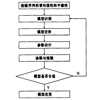• 关于时间序列模型的比较准确的描述，可以通过这个pdf来学习一下传统的经典时间序列模型
• 第五章 时间序列模型的分析 第一节 时间序列模型简介 时间序列模型(time series model) 第二节 平稳时间序列模型 时序图 平稳时间序列的意义 例 5-1 白噪声时间序列(White noise time series) 一移动平均过程(MA ) ...
• ## 时间序列模型 （一）：模型概述

万次阅读 多人点赞 2019-04-21 21:47:12
时间序列的其它博文系列： 时间序列模型 （一）：模型概述...时间序列模型 （六）：平稳时间序列模型 ：自回归AR 、移动平均 MA 、ARMA 模型 时间序列模型 （七）： 时间序列建模的基本步骤 目录 时间序列的不同...
时间序列的其它博文系列：

时间序列模型 （一）：模型概述

时间序列模型 （二）：移动平均法

时间序列模型 （三）：指数平滑法

时间序列模型 （四）：差分指数平滑法、 自适应滤波法v

时间序列模型 （五）： 趋势外推预测方法

时间序列模型 （六）：平稳时间序列模型 ：自回归AR 、移动平均 MA 、ARMA 模型

时间序列模型 （七）： 时间序列建模的基本步骤

目录

时间序列的不同分类

确定性时间序列分析方法概述

三种时间序列模型

时间序列的不同分类

时间序列是按时间顺序排列的、随时间变化且相互关联的数据序列。分析时间序 列的方法构成数据分析的一个重要领域，即时间序列分析。 时间序列根据所研究的依据不同，可有不同的分类。

1．按所研究的对象的多少分，有一元时间序列和多元时间序列。

2．按时间的连续性可将时间序列分为离散时间序列和连续时间序列两种。

3．按序列的统计特性分，有平稳时间序列和非平稳时间序列。如果一个时间序列的概率分布与时间t无关，则称该序列为严格的（狭义的）平稳时间序列。如果序列的 一、二阶矩存在，而且对任意时刻t满足：

（1）均值为常数

（2）协方差为时间间隔  的函数。 则称该序列为宽平稳时间序列，也叫广义平稳时间序列。我们以后所研究的时间序列主 要是宽平稳时间序列。

4．按时间序列的分布规律来分，有高斯型时间序列和非高斯型时间序列。

确定性时间序列分析方法概述

时间序列预测技术就是通过对预测目标自身时间序列的处理，来研究其变化趋势 的。一个时间序列往往是以下几类变化形式的叠加或耦合。 我们常认为一个时间序列可以分解为以下四大部分：

（1）长期趋势变动。它是指时间序列朝着一定的方向持续上升或下降，或停留在 某一水平上的倾向，它反映了客观事物的主要变化趋势。

（2）季节变动。

（3）循环变动。通常是指周期为一年以上，由非季节因素引起的涨落起伏波形相 似的波动。

（4）不规则变动。通常它分为突然变动和随机变动。

三种时间序列模型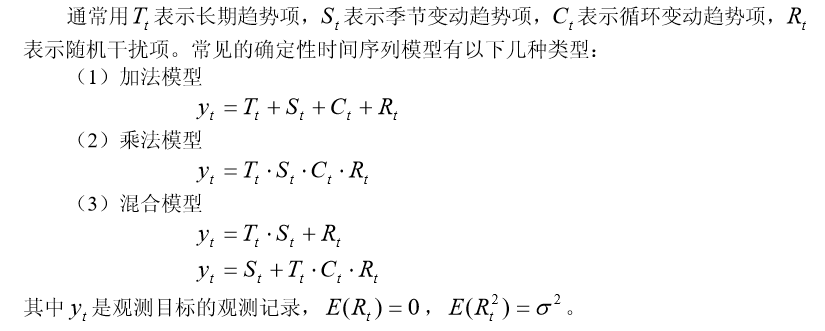如果在预测时间范围以内，无突然变动且随机变动的方差  较小，并且有理由认 为过去和现在的演变趋势将继续发展到未来时，可用一些经验方法进行预测。

时间序列的其它博文系列：

时间序列模型 （一）：模型概述

时间序列模型 （二）：移动平均法

时间序列模型 （三）：指数平滑法

时间序列模型 （四）：差分指数平滑法、 自适应滤波法v

时间序列模型 （五）： 趋势外推预测方法

时间序列模型 （六）：平稳时间序列模型 ：自回归AR 、移动平均 MA 、ARMA 模型

时间序列模型 （七）： 时间序列建模的基本步骤

展开全文• ## 算法模型---时间序列模型

万次阅读 多人点赞 2018-01-16 09:04:58
1、时间序列时间序列是时间间隔不变的情况下收集的不同时间点数据集合，这些集合被分析用来了解长期发展趋势及为了预测未来。...常用的时间序列模型有AR模型、MA模型、ARMA模型和ARIMA模型等。2、时间
文章来源
时间序列
时间序列是时间间隔不变的情况下收集的不同时间点数据集合，这些集合被分析用来了解长期发展趋势及为了预测未来。
时间序列与常见的回归问题的不同点在于:
1、时间序列是跟时间有关的;而线性回归模型的假设：观察结果是独立的在这种情况下是不成立的。
2、随着上升或者下降的趋势，更多的时间序列出现季节性趋势的形式；
常用的时间序列模型有AR模型(Autoregressive model:自回归模型)、MA模型(moving average model:滑动平均模型)、ARMA模型(Auto-Regressive and Moving Average Model:自回归滑动平均模型)和ARIMA模型(Autoregressive Integrated Moving Average Model:自回归积分滑动平均模型)等。
时间序列的预处理(使数据平稳化和随机化)
拿到一个观察值序列之后，首先要对它的平稳性和纯随机性进行检验，这两个重要的检验称为序列的预处理。根据检验的结果可以将序列分为不同的类型，对不同类型的序列我们会采用不同的分析方法。
平稳化处理
平稳 就是围绕着一个常数上下波动且波动范围有限，即有常数均值和常数方差。如果有明显的趋势或周期性，那它通常不是平稳序列。序列平稳不平稳，一般采用三种方法检验：
时序图检验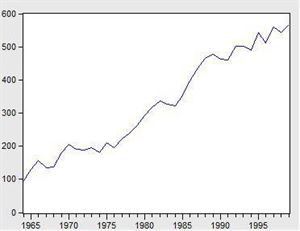看看上面这个图，很明显的增长趋势，不平稳。
利用自相关系数和偏相关系数
自相关系数和偏相关系数的概念可参考《算法模型— 概率论基础—相关系数相关》
下面是不平稳数据的自相关和偏相关系数的一种情形。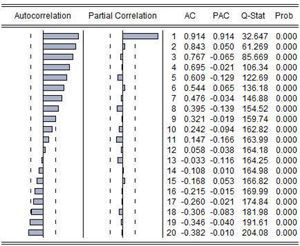左边第一个为自相关图（Autocorrelation），第二个偏相关图(Partial Correlation)。
平稳的序列的自相关图和偏相关图要么拖尾，要么是截尾。截尾就是在某阶之后，系数都为 0 。怎么理解呢，看上面偏相关的图，当阶数为 1 的时候，系数值还是很大， 0.914;二阶长的时候突然就变成了 0.050. 后面的值都很小，认为是趋于 0 ，这种状况就是截尾。什么是拖尾，拖尾就是有一个缓慢衰减的趋势，但是不都为 0 。
自相关图既不是拖尾也不是截尾。以上的图的自相关是一个三角对称的形式，这种趋势是单调趋势的典型图形，说明这个序列不是平稳序列。
平稳序列的自相关系数会快速衰减。
单位根检验
单位根检验是指检验序列中是否存在单位根，如果存在单位根就是非平稳时间序列。
单位根检验：ADF是一种常用的单位根检验方法，他的原假设为序列具有单位根，即非平稳，对于一个平稳的时序数据，就需要在给定的置信水平上显著，拒绝原假设。ADF只是单位根检验的方法之一，如果想采用其他检验方法，可以安装第三方包arch，里面提供了更加全面的单位根检验方法，个人还是比较钟情ADF检验。以下为检验结果，其p值大于0.99，说明并不能拒绝原假设。
利用差分将序列数据转换为平衡序列
差分可以将数据转换为平稳序列。
一阶差分指原序列值相距一期的两个序列值之间的减法运算；k阶差分就是相距k期的两个序列值之间相减。如果一个时间序列经过差分运算后具有平稳性，则该序列为差分平稳序列，可以使用ARIMA模型进行分析。
确定不平稳后，依次进行1阶、2阶、3阶…差分，直到平稳为止。
随机化处理
对于纯随机序列，又称白噪声序列，序列的各项数值之间没有任何相关关系，序列在进行完全无序的随机波动，可以终止对该序列的分析。白噪声序列是没有信息可提取的平稳序列。对于平稳非白噪声序列，它的均值和方差是常数。通常是建立一个线性模型来拟合该序的发展，借此提取该序列的有用信息。ARMA模型是最常用的平稳序列拟合模型。
平稳时间序列建模
某个时间序列经过预处理，被判定为平稳非白噪声序列，就可以进行时间序列建模。
建模步骤：
（1）计算出该序列的自相关系数（ACF）和偏相关系数（PACF）；
（2）模型识别，也称模型定阶。根据系数情况从AR§模型、MA(q)模型、ARMA(p，q)模型、ARIMA（p，d，q）模型中选择合适模型，其中p为自回归项，d为差分阶数，q为移动平均项数。
若平稳序列的偏相关函数是截尾的，而自相关函数是拖尾的，可断定序列适合AR模型；若平稳序列的偏相关函数是拖尾的，而自相关函数是截尾的，则可断定序列适合MA模型；若平稳序列的偏相关函数和自相关函数均是拖尾的，则序列适合ARMA模型。（截尾是指时间序列的自相关函数（ACF）或偏自相关函数（PACF）在某阶后均为0的性质（比如AR的PACF）；拖尾是ACF或PACF并不在某阶后均为0的性质（比如AR的ACF）。）
（3）估计模型中的未知参数的值并对参数进行检验；
（4）模型检验；
（5）模型优化；
（6）模型应用：进行短期预测。
混合自回归移动平均过程（公式表达）
差分方程
所谓差分方程即将变量 $y_t$与它的滞后期联系起来的表达式。
研究变量在第t期的值记为$y_t$。假定给出的动态方程将变量y第t期的值与另外的变量$w_t$以及y的前一期联系起来:
$y_t=ϕy_{t−1}+w_t$
上述称为一阶差分方程是因为仅仅只有变量的一阶滞后$(y_t−1)$出现在方程中。
移动平均
“移动平均”的含义源于$Y_t$是最近两期的$\epsilon$的加权平均。
令${\epsilon_t}$是一个白噪声序列。
$Y_t=μ+\epsilon_t+θ\epsilon_{t−1}$
其中μ和θ可以是任意的常数。这个时间序列称为一阶移动平均过程，记为$MA(1)$。
自回归过程
一阶自回归过程
一个一阶自回归，记作$AR(1)$满足下面的差分方程
$Y_t=c+ϕY_{t−1}+\epsilon_t$
${\epsilon_t}$是一个白噪声序列。
p阶自回归过程
一个p阶自回归，记作$AR(p)$满足下式
$Y_t=c+ϕ_1Y_{t−1}+ϕ_2Y_{t−2}+...+ϕ_pY{t−p}+\epsilon_t$
混合自回归移动平均过程
一个$ARMA(p,q)$过程包括自回归和移动平均项：
$Y_t=c+ϕ_1Y_{t−1}+ϕ_2Y_{t−2}+...+ϕ_pY{t−p}+\epsilon_t+θ_1ϵ_{t−1}+θ_2ϵ{t−2}+...+θ_qϵ_{t−q}$
利用statsmodels库实现时间序列的分析处理
Time Series analysis tsa
statsmodels.tsa.arima_model.ARIMA
AR(I)MA时间序列建模过程——步骤和python代码
Seasonal ARIMA with Python
Python 3中使用ARIMA进行时间序列预测的指南
Python_Statsmodels包_时间序列分析_ARIMA模型
时间序列实战（一）
How to Make Predictions for Time Series Forecasting with Python
How to Use and Remove Trend Information from Time Series Data in Python
时间序列分析之ARIMA上手-Python
[python] 时间序列分析之ARIMA
python中有专门的库statsmodels可以用来作时间序列分析，但spark中则没有现成的。
#-*- coding: utf-8 -*-
import matplotlib.pyplot as plt
import pandas as pd
data=pd.read_csv('./HIS_MONTH.csv')
b=data['fData']
plt.plot(data['fData'])
plt.show()

数据结构如下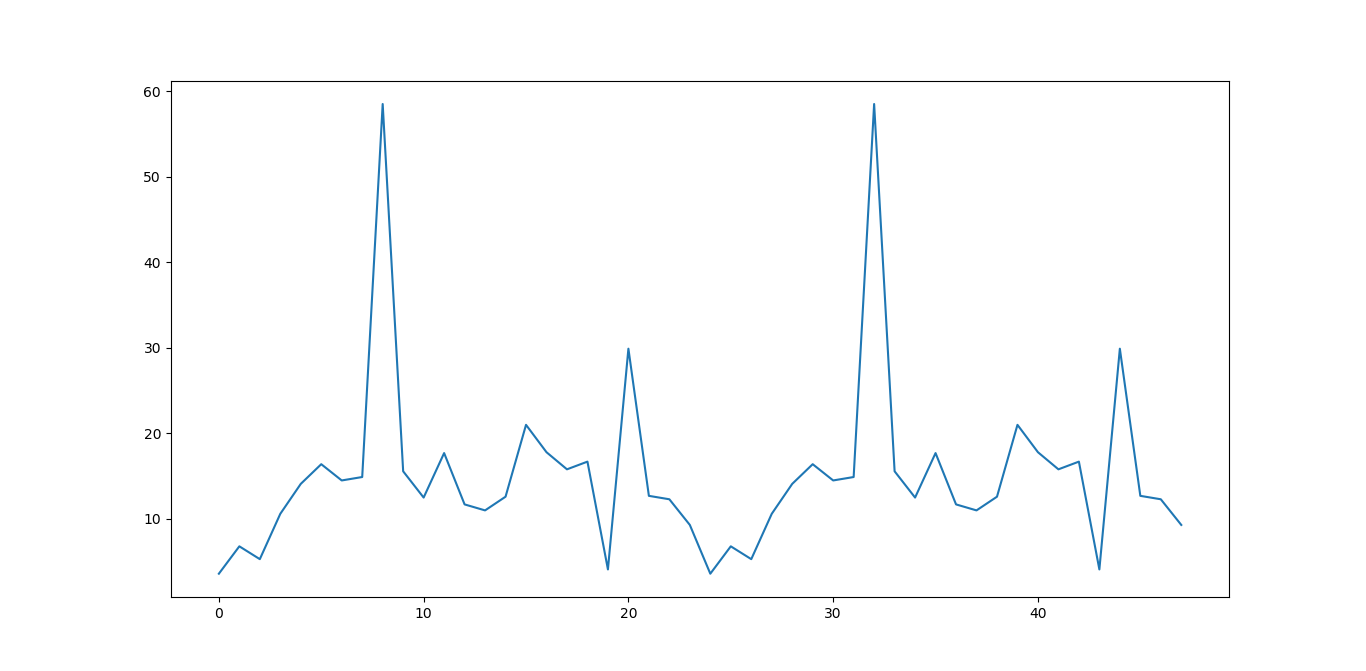#自相关图
from statsmodels.graphics.tsaplots import plot_acf
plot_acf(data['fData']).show()

如下所示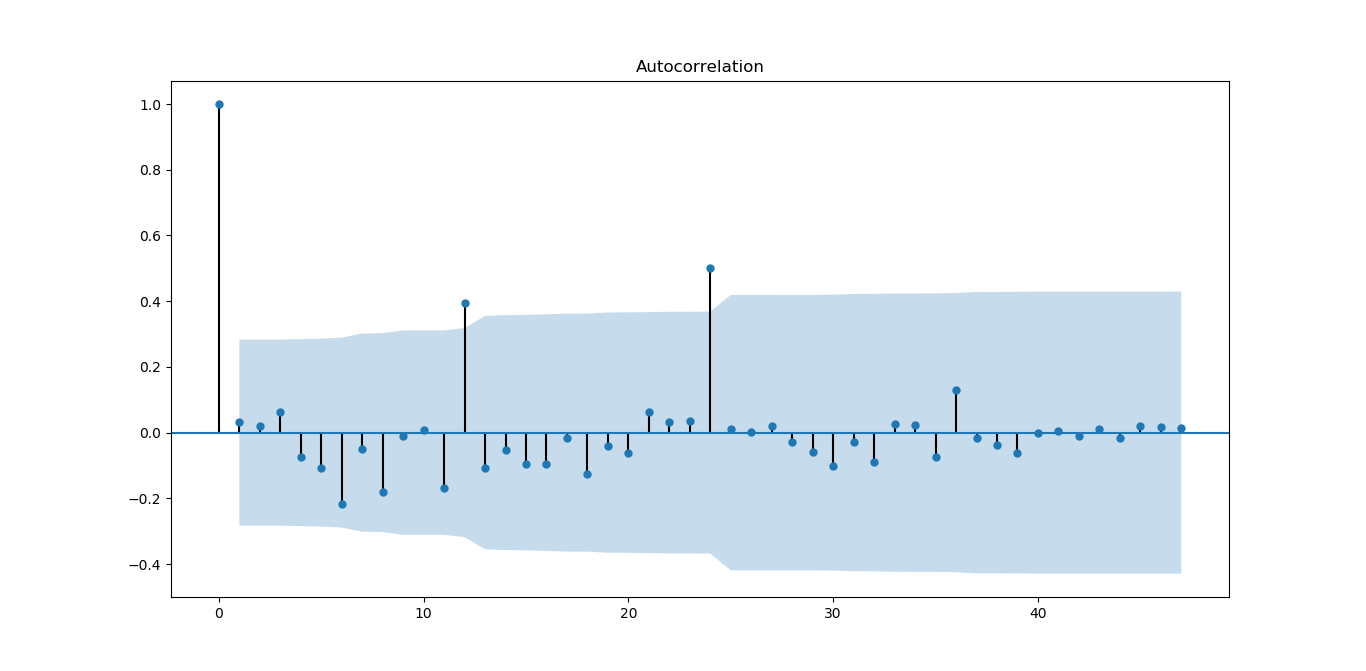从图上可以自相关系数基本是拖尾的，稍做调整就可以使用。
#偏自相关图
from statsmodels.graphics.tsaplots import plot_pacf
plot_pacf(data['fData']).show()

如下图所示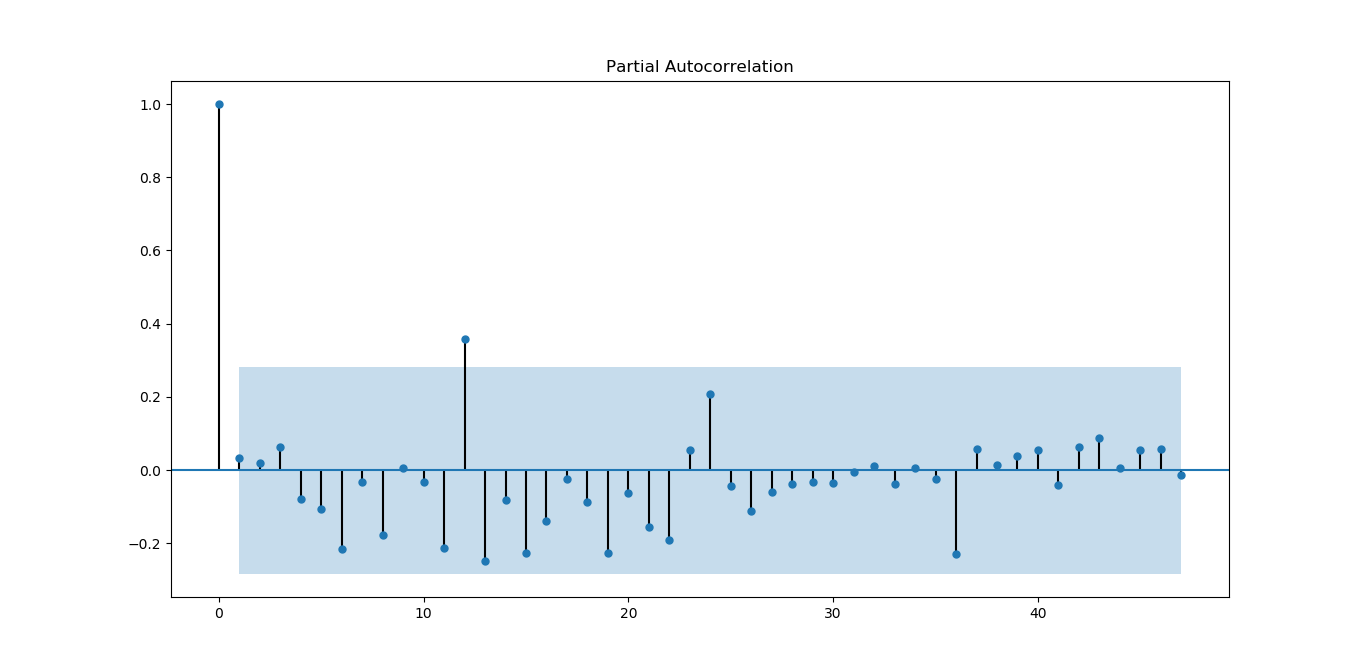偏相关系数也基本符合拖尾。
#平稳性检测
from statsmodels.tsa.stattools import adfuller as ADF
print('原始序列的ADF检验结果为：')
print(ADF(data['fData']))
#返回值依次为adf、pvalue、usedlag、nobs、critical values、icbest、regresults、resstore

如下所示
原始序列的ADF检验结果为：
(-6.561077625309946, 8.378411469638636e-09, 0L, 47L, {'5%': -2.925338105429433, '1%': -3.5778480370438146, '10%': -2.6007735310095064}, 273.5266547319719)

p值远小于0.05，拒绝原假设（原假设认为存在单位根），所以该序列为平衡时间序列。
#白噪声检验
from statsmodels.stats.diagnostic import acorr_ljungbox
print('差分序列的白噪声检验结果为：')
print(acorr_ljungbox(data['fValueData'], lags=1))

结果如下
差分序列的白噪声检验结果为：
(array([0.05198092]), array([0.81965149]))

P=0.81965149，统计量的P值大于显著性水平0.05，则接受原假设（原假设认为为白噪声序列）。
下面对原序列做一阶差分运算
#差分后的结果
D_data = data['fValueData'].diff().dropna()
D_data.columns = ['fValueData']
#时序图
D_data.plot()

结果如下：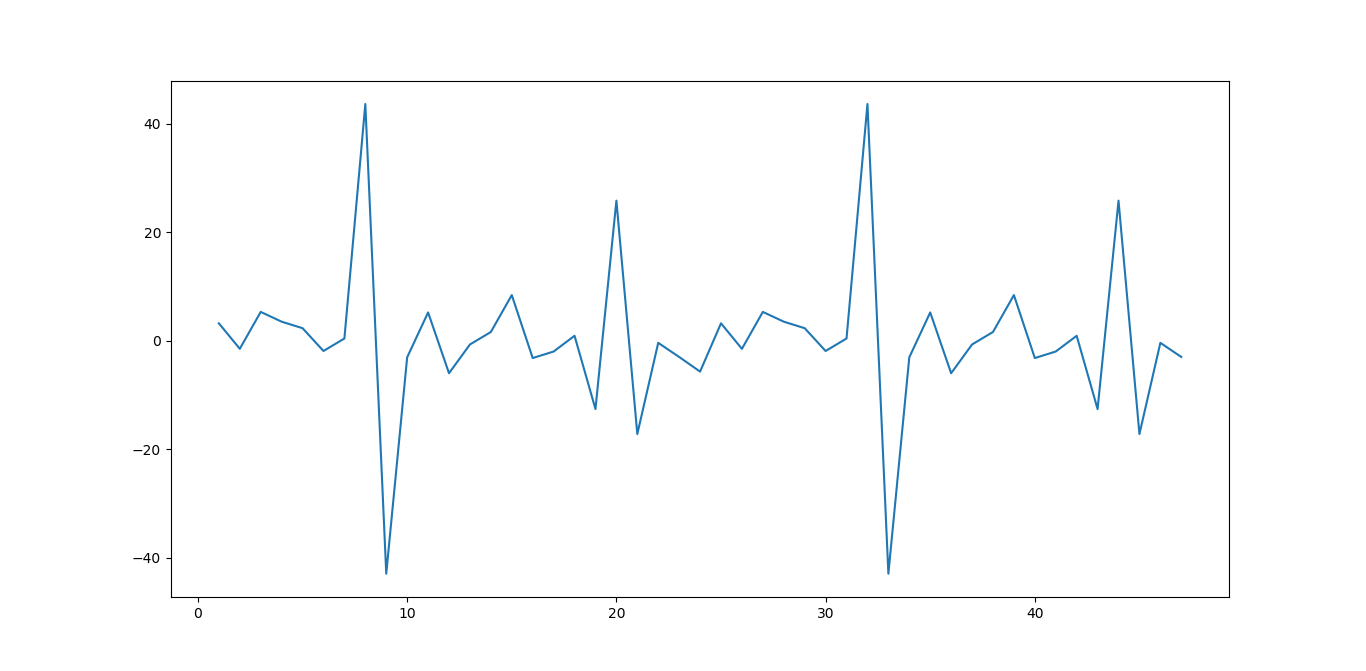从图上看，一阶差分后，感觉数据分布更对称为随机了。
#自相关图
plot_acf(D_data).show()
plt.show()

结果如下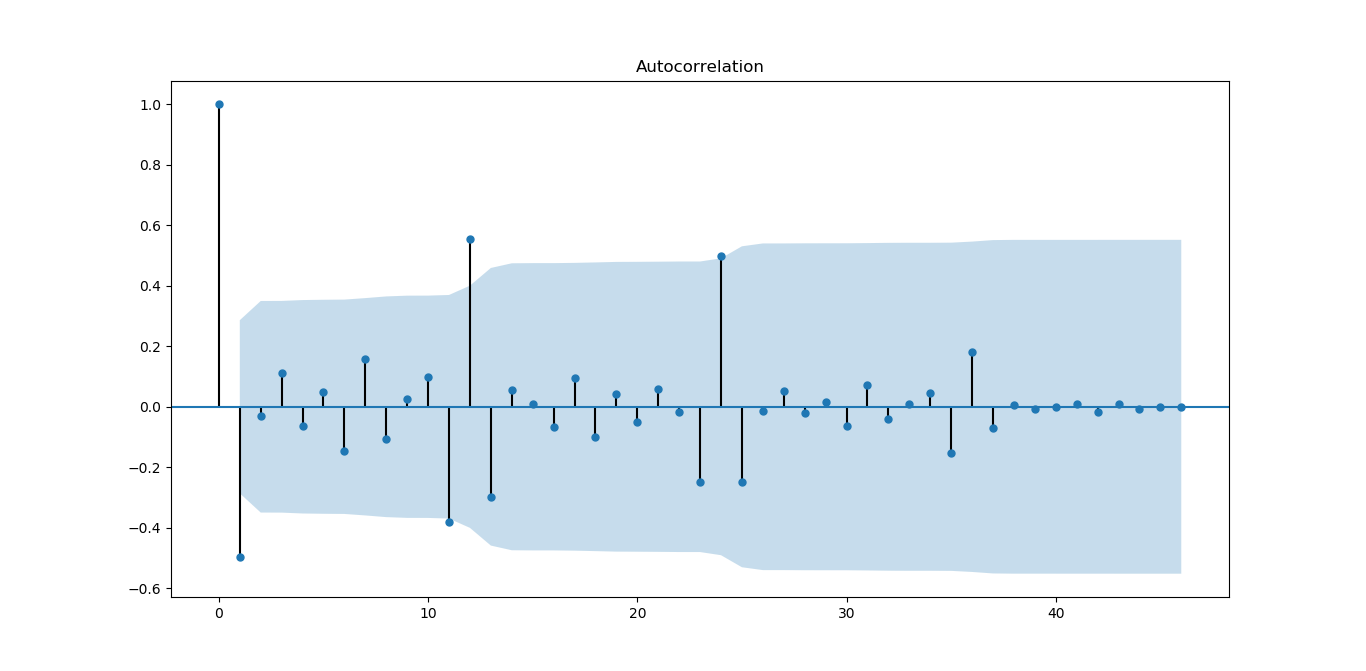从图上看，一阶差分对数据有改善，振荡衰减更明显
#偏自相关图
plot_pacf(D_data).show()

结果如下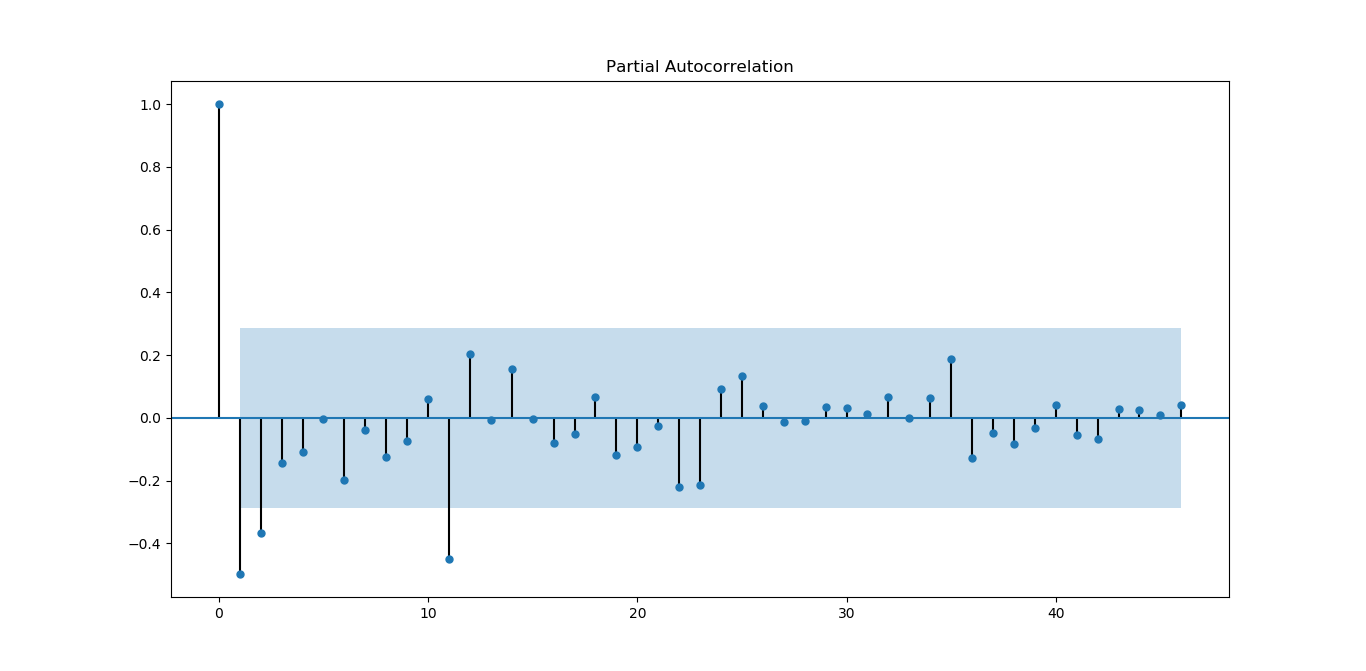从图上看，偏相关系数虽然没有自相关系数好，但基本能满足平稳序列的要求。
#平稳性检测
print('差分序列的ADF检验结果为：')
print( ADF(D_data))

结果如下
差分序列的ADF检验结果为：
(-4.595500765524432, 0.0001316587309452837, 10L, 36L, {'5%': -2.9459512825788754, '1%': -3.626651907578875, '10%': -2.6116707716049383}, 270.35268975914374)

p值远小于0.05，拒绝原假设（原假设认为存在单位根），所以该序列为平衡时间序列。
#白噪声检验
from statsmodels.stats.diagnostic import acorr_ljungbox
#返回统计量和p值
print('差分序列的白噪声检验结果为：')
print(acorr_ljungbox(D_data, lags=1))

结果如下：
差分序列的白噪声检验结果为：
(array([12.35619393]), array([0.00043953]))

P=0.00043953，统计量的P值小于显著性水平0.05，则拒绝原假设（原假设认为为白噪声序列），所以一阶差分后该序列为非白噪声序列，有进一步分析的价值。
Holt-Winters模型原理及python实现
本部分来源，也可以参考该文
原理分析
公式前面也写过，这里再表述一下，有时间再来整理
假设随机时间序列为：
$x_t(t=1,2,\ldots,N,N=n)$
时间序列数据一般有以下几种特点：1.趋势(Trend)  2. 季节性(Seasonality)。
趋势描述的是时间序列的整体走势，比如总体上升或者总体下降。下图所示的时间序列是总体上升的：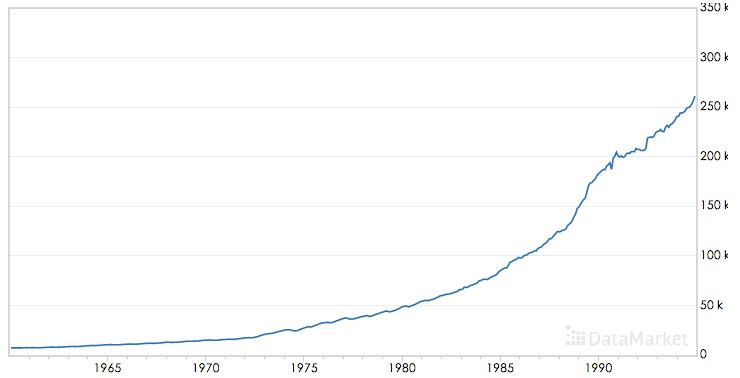季节性描述的是数据的周期性波动，比如以年或者周为周期，如下图：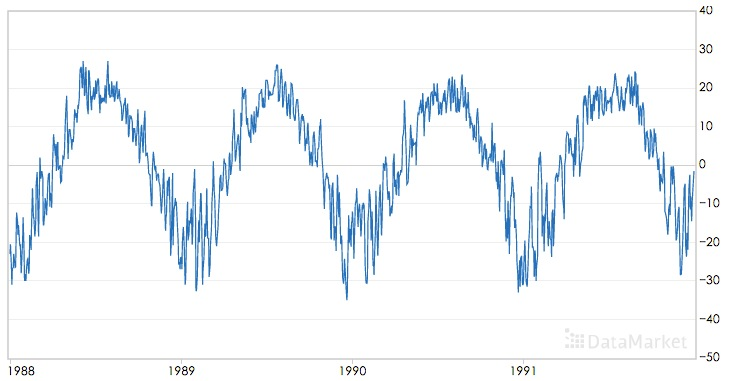三次指数平滑算法可以对同时含有趋势和季节性的时间序列进行预测，该算法是基于一次指数平滑和二次指数平滑算法的。
移动平均(The simple moving average (MA))
直观上,最简单的平滑时间序列的方法是实现一个无权重的移动平均,目前已知的方法是用窗口函数,平滑统计量 $S_t$就是最近k个观察值的均值。公式如下:
$s_t=\frac{1}{k} \sum_{n=0}^{k-1} x_{t-n}=\frac{x_t+x_{t-1}+\ldots + x_{t-k+1}}{k}=s_{t-1}+\frac{x_t -x_{t-k}}{k}$
这样的方法存在明显的缺陷,当k比较小时,预测的数据平滑效果不明显,而且突出反映了数据最近的变化;当k较大时,虽然有较好的平滑效果,但是预测的数据存在延迟。而且最少需要k个值(窗口有限)。
加权移动平均
一种稍微复杂的方法是先选择一组权重因子来计算加权移动平均
${\omega_1,\omega_2,\ldots,\omega_k},满足\sum_{n=1}^{k}\omega_n=1$
然后用这些权重来计算这些平滑统计量:
$s_t=\sum_{n=1}^{k}\omega_n x_{t-n+1}=\omega_1x_t+\omega_2 x_{t-1}+\ldots+\omega_{k}x_{t-k+1}$
在实践中,通常在选择权重因子时,赋予时间序列中的最新数据更大的权重,并减少对旧数据的权重。这个方法也需要最少k个值,并且计算复杂。
简单指数平滑法
幸运地是有一种方法可以避免上述问题,它叫做指数平滑法。最简单的指数平滑法如下:
$s_i = \alpha x_i + (1-\alpha)s_{i-1}$
其中$\alpha$是平滑因子,$0 < \alpha < 1$。$s_i$是当前时刻的平滑值。换句话说,平滑统计值$S_i$是当前统计值$X_t$与上一时间平滑值$S_{i-1}$加权平均。这个简单指数平滑是很容易被应用的,因为只要有两个观察值就能计算了。这里α的选取,我们可以采用最小二乘来决定$\alpha$(最小化$(s_t-x_t)^2$)。
一次指数平滑算法进行预测的公式为：
$x_{i+h}=s_i$
其中i为当前最后的一个数据记录的坐标，亦即预测的时间序列为一条直线，不能反映时间序列的趋势和季节性。
为什么被称为“指数”平滑法
从它的递推公式就能发现:
简单指数平滑法适用于没有总体趋势的时间序列。如果用来处理有总体趋势的序列,平滑值将往往滞后于原始数据,除非α的值接近1,但这样一来就会造成不够平滑。
二次指数平滑（HoltWinters-无季节趋势）
为了解决上述问题,于是引出了二次指数平滑,能够保留总体趋势信息。因为将指数平滑应用了两次,所以被称为二次指数平滑。与简单指数平滑相比,二次指数平滑加入了时间趋势统计量$t_i$,公式如下:
$s_i=\alpha x_i + (1-\alpha)(s_{i-1}+t_{i-1})\\ t_i=\beta (s_i-s_{i-1}) + (1-\beta)t_{i-1}$
$s_i$添加了与一次指数平滑相比，添加了一个新的趋势项$t_i$；而$t_i$的表达式与一次指数平滑形式上是一样的，只不过用将$x_i$换成$(s_i-s_{i-1})$,趋势可以想像成增量，就是在此刻与上一刻的差值，趋势当然应该用平滑后的数据的差值；同时将一次平滑中的$s_{i-1}$换成$t_{i-1}$
二次指数平滑的预测公式为
$x_{i+h}=s_i+h \ t_i$
二次指数平滑的预测结果是一条斜的直线
三次指数平滑
三次指数平滑将时间序列的季节性这一特征也考虑进去了。
季节性被定义为时间序列数据的趋势,它表现出每一个周期重复自身的行为,就像任何周期函数一样。“季节”这个词用来表示行为每隔时间段L就开始自我重复。在自然界中有不同类型的季节性“累加性”(additive)和“累乘性“(multiplicative),就像加法和乘法是数学的基本运算。
如果每个12月都比每个11月多卖出1000套公寓,我们就说这样的季节趋势是“累加性”的。可以用绝对增⻓来表示。如果我们在夏季比冬季多卖出10%的公寓,那么季节趋势在自然中是“累乘性”的。
累乘性公式如下:
下面的式子中$k$表示季节频率，这个还需要查资料进行理解
$s_i=\alpha \frac{x_i }{p_{i-k}} +(1-\alpha)(s_{i-1}+t_{i-1})\\ t_i=\beta (s_i-s_{i-1}) + (1-\beta)t_{i-1}\\ p_i=\gamma \frac{x_i}{s_i} + (1-\gamma)p_{t-k}\\$
上式中k为周期， 累乘三次指数平滑的预测公式为：
$x_{i+h}=(s_i+h\ t_i)p_i-k+(h\ mod\ k)$
或者
$x_{i+h}=(s_i+h\ t_i)p_{i-k+1+(h-1) \ mod \ k}$
两个公式还需要确认下
累乘性公式初始值的计算
$s_k=\frac{1}{k} (s_1+s_2+\cdots+s_k)\\ t_k=\frac{1}{k}\left[ \frac{x_{k+1}-x_1}{k}+\frac{x_{k+2}-x_2}{k}+\cdots+\frac{x_{k+k}-x_k}{k} \right]\\ p_1=\frac{x_1}{s_k},p_2=\frac{x_2}{s_k},\cdots,p_k=\frac{x_k}{s_k}$
累加性公式如下:
$s_i=\alpha (x_i-p_{i-k} )+(1-\alpha)(s_{i-1}+t_{i-1})\\ t_i=\beta (s_i-s_{i-1}) + (1-\beta)t_{i-1}\\ p_i=\gamma (x_i-s_i) + (1-\gamma)p_{i-k}\\ x_{i+h}=s_i+hb_i + p_{i-k+h \ mod \ k}$
或者
$x_{i+h}=s_i+hb_i + p_{i-k+1+(h-1) \ mod \ k}$
两个公式还需要确认下
累加性公式初始值的计算
$s_k=\frac{1}{k} (s_1+s_2+\cdots+s_k)\\ t_k=\frac{1}{k}\left[ \frac{x_{k+1}-x_1}{k}+\frac{x_{k+2}-x_2}{k}+\cdots+\frac{x_{k+k}-x_k}{k} \right]\\ p_1=x_1-s_k,p_2=x_2-s_k,\cdots,p_k=x_k-s_k$
其中 $\alpha$是数据平滑因子, $0 < \alpha < 1$;$\beta$是趋势平滑因子,$0 < \beta < 1$; $\gamma$是季节改变平滑因子$0 < \gamma < 1$。
$\alpha，\beta，\gamma$的值都位于[0,1]之间，可以多试验几次以达到最佳效果。
$s,t,p$初始值的选取对于算法整体的影响不是特别大，通常的取值为$s_0=x_0,t_0=x_1-x_0$,累加时$p=0$,累乘时$p=1$.
对三次指数平滑法而言,我们必须初始化一个完整的“季节”$p_i$的值,不过我们可以简单地设置为全1(针对累乘式)或全0(针对累加式)。只有当序列的⻓度较短时,我们才需要慎重考虑初始值的选取。
我们这里讲的Holt-Winters模型就是三次指数平滑法。哇,终于切入正题了。
所有的指数平滑法都要更新上一时间步⻓的计算结果,并使用当前时间步⻓的数据中包含的新信息。它们通过“混合”新信息和旧信息来实现,而相关的新旧信息的权重由一个可调整的拌和参数来控制。各种方法的不同之处在于它们跟踪的量的个数和对应的拌和参数的个数。三次指数平滑法,功能最强大,既能体现趋势性又能体现季节性,所以三次指数平滑法的参数最多,有三个。
下图为使用累加三次指数平滑进行预测的效果：其中红色为源时间序列，蓝色为预测的时间序列，$\alpha，\beta，\gamma$的取值为0.45，0.2，0.95：下图为累乘三次指数平滑进行预测的效果，$\alpha，\beta，\gamma$的取值为0.4，0.05，0.9：可以看到三次指数平滑算法可以很好的保存时间序列数据的趋势和季节性信息，在International Airline Passengers数据集上累乘平滑指数算法的效果更好。
python代码实现
我们知道HoltWinters模型有三个可调参数,我们的目的就是训练出有效的α,β, γ
。我们有两种方法,一种就是自己取值来试试,一种就是采用数值优化的思想,比如
前面我们提到的最小二乘来最小化误差来求参数(注意不一定能全局收敛!这个问题
实在是让人头痛。。。)我们就采用最小二乘法(L-BFGS)。
RMSE的实现
statsmodels中的holtwinters
statsmodels.tsa模块
statsmodels.tsa.holtwinters源码
statsmodels.tsa.holtwinters.ExponentialSmoothing
ExponentialSmoothing - 指数平滑算法
基于SVM作短期时间序列的预测
传统的做法是提取1、2、3、4、5、7、9、13个单位时间的数据作为特征进行预测；
举个例子进行分析，比如每天都有口香糖的销量，那么如何通过几周的数据预测明天的数据，
就可以选择前1、2、3、4、5、7、14天的数据作为特征，从而预测明天的数据，
通过构建特征，再选择核函数进行预测，其中调参的参数尽量要进行最优化，
参考方法：如果选择RBF核函数，那么其中就会有三个参数，固定两个，然后不停的优化另外一个，直到得到最优解。
具体应用的例子：
（1）SVM预测风场：http://wenku.baidu.com/link?url=SCCIJJe8tXLbTjLMZ81x5Qy6elsceAKIOwtkZ0QxfSCQQ4KaWKwo8Biepjs3Ss2LJ2ewhisNR0ixrDY4kV1Rd7BcqWRenuTaG85K80E-30y
（2）SVM预测股票指数：基于SVM修正的模糊时间序列模型在沪指预测中的应用
（3）SVM预测时间序列其他方面：http://www.docin.com/p-233353900.html
LSTM模型分析及对时序数据预测的具体实现（python实现）
来源：2017年09月30日 10:28:08
如何用LSTM自编码器进行极端事件预测？(含Python代码)
时间序列的并行实现
spark里面的库是没有时间序列算法的，但是国外有人已经写好了相应的算法。其github网址是:https://github.com/sryza/spark-timeseries
sryza/spark-timeseries
Spark-TimeSeries使用方法
A New Library for Analyzing Time-Series Data with Apache Spark
【Spark Summit East 2017】使用Spark进行时间序列分析
基于小波变换的时间序列预测，Python实现，来自雪球
清华AIOps算法：KPI聚类
其他资料
时间序列挖掘-预测算法-三次指数平滑法(Holt-Winters)
Holt-Winters原理和初始值的确定


展开全文数据
• 时间序列模型初步致力于为大家提供学习、参考最实用的资源，对时间序列模型初步有需要的朋友，赶快来下载...该文档为时间序列模型初步，是一份很不错的参考资料，具有较高参考价值，感兴趣的可以下载看看
• 时间序列的其它博文系列： 时间序列模型 （一）：模型...时间序列模型 （六）：平稳时间序列模型 ：自回归AR 、移动平均 MA 、ARMA 模型 时间序列模型 （七）： 时间序列建模的基本步骤 这里的平稳是指宽平稳，...
时间序列的其它博文系列：

时间序列模型 （一）：模型概述

时间序列模型 （二）：移动平均法

时间序列模型 （三）：指数平滑法

时间序列模型 （四）：差分指数平滑法、 自适应滤波法v

时间序列模型 （五）： 趋势外推预测方法

时间序列模型 （六）：平稳时间序列模型 ：自回归AR 、移动平均 MA 、ARMA 模型

时间序列模型 （七）： 时间序列建模的基本步骤

这里的平稳是指宽平稳，其特性是序列的统计特性不随时间的平移而变化，即均值和协方差不随时间的平移而变化。

自回归模型（Auto Regressive Model）简称 AR 模型，移动平均模型（Moving Average Model）简称 MA 模型，

自回归移动平均模型（Auto Regressive Moving Average Model）简称 ARMA 模型。

下面的  为零均值（即中心化处理的）平稳序列。

目录

一般自回归模型 AR(n)

白噪声序列

移动平均模型 MA(m)

自回归移动平均模型

ARMA 模型的特性

AR(1)系统的格林函数

ARMA (2,1)系统的格林函数 的隐式

逆函数和可逆性

一般自回归模型 AR(n)

白噪声序列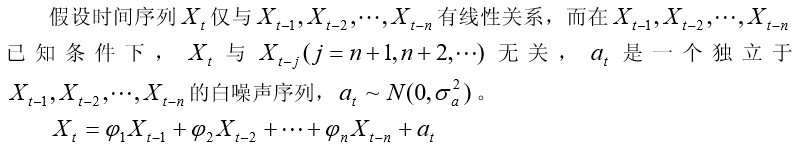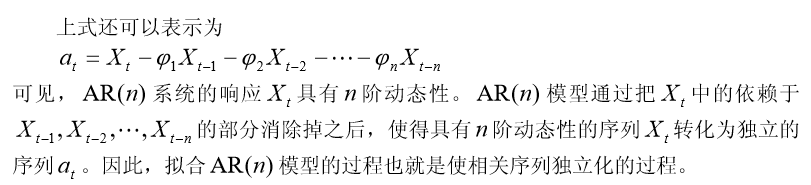移动平均模型 MA(m)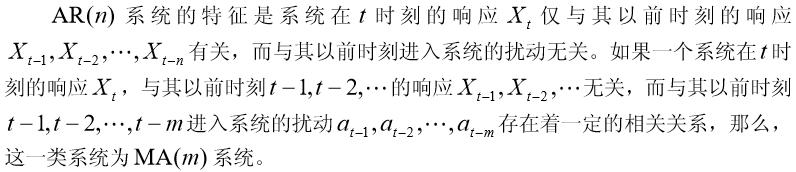自回归移动平均模型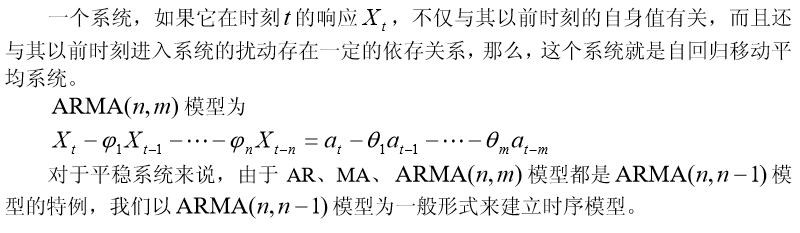ARMA 模型的特性

在时间序列的时域分析中，线性差分方程是极为有效的工具。事实上，任何一个 ARMA 模型都是一个线性差分方程。

AR(1)系统的格林函数

格林函数就是描述系统记忆扰动程度的函数。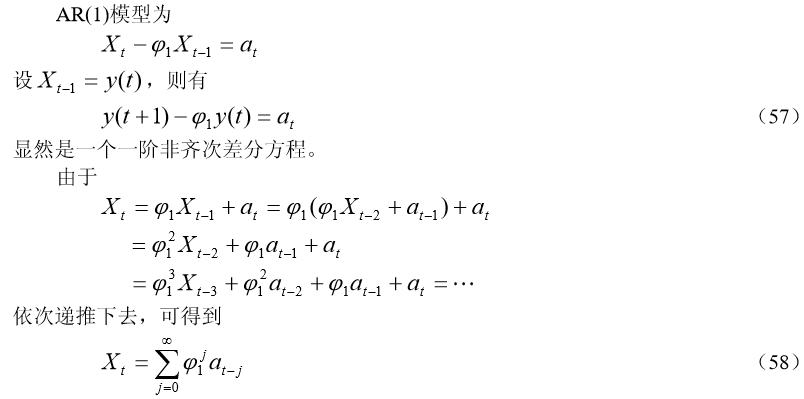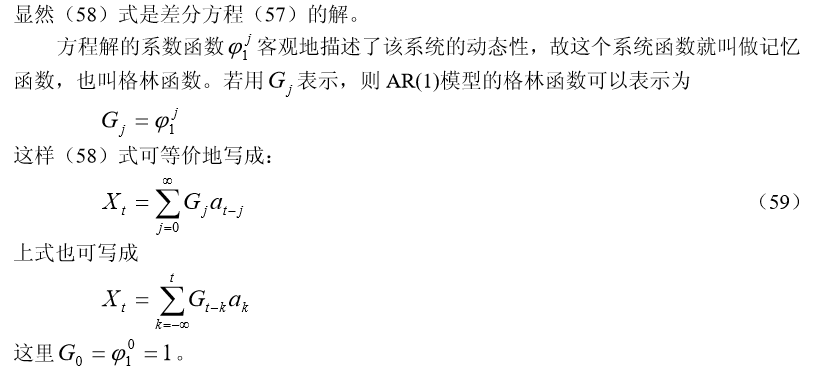后移算子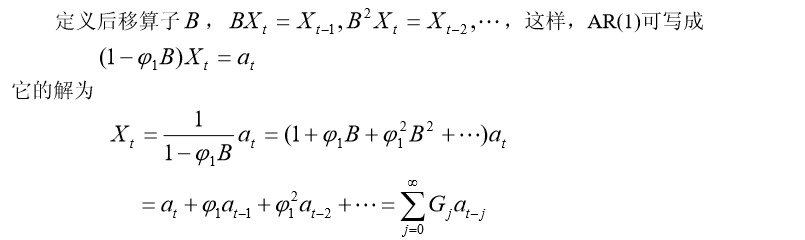由于格林函数就是差分方程解的系数函数，格林函数的意义可概括如下：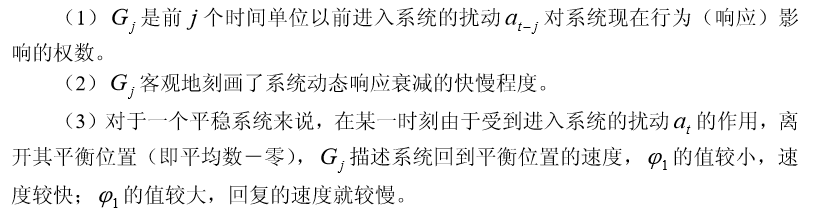ARMA (2,1)系统的格林函数 的隐式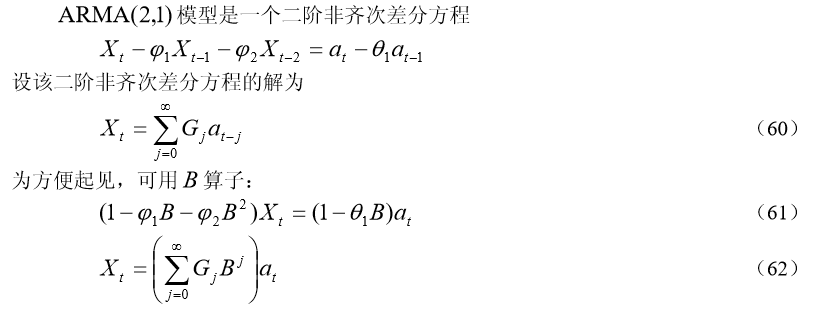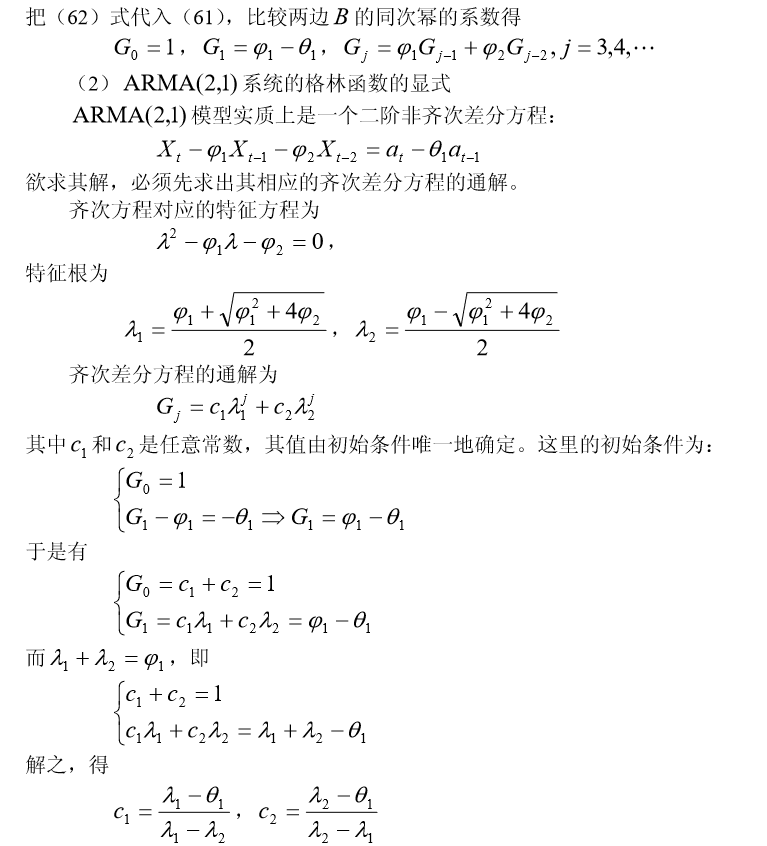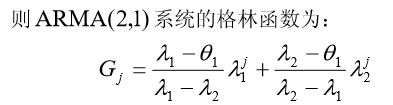逆函数和可逆性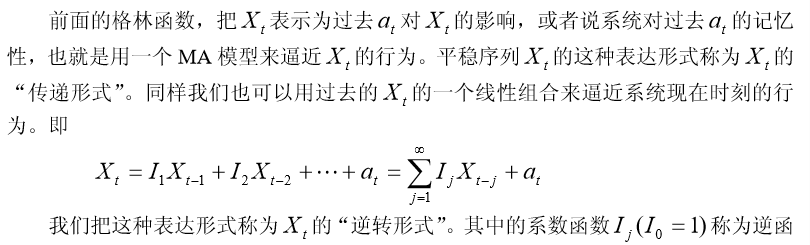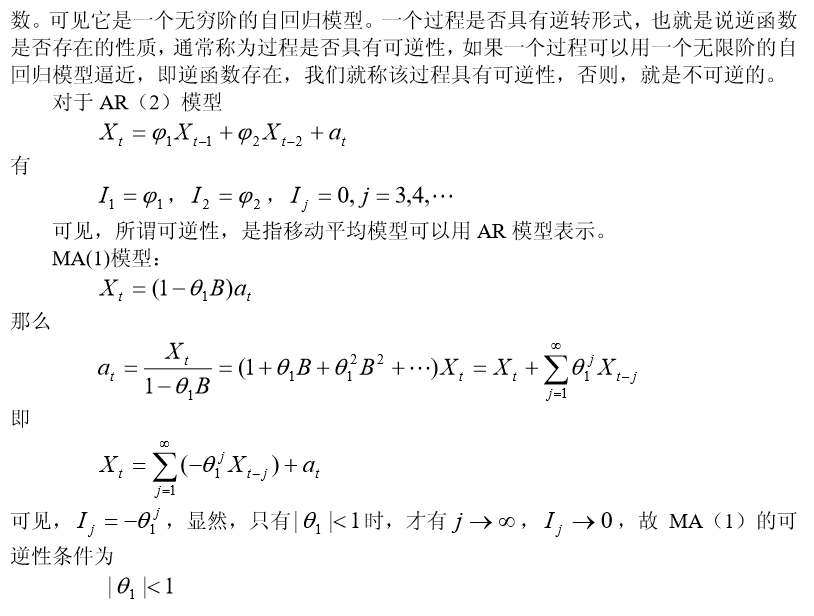时间序列的其它博文系列：

时间序列模型 （一）：模型概述

时间序列模型 （二）：移动平均法

时间序列模型 （三）：指数平滑法

时间序列模型 （四）：差分指数平滑法、 自适应滤波法v

时间序列模型 （五）： 趋势外推预测方法

时间序列模型 （六）：平稳时间序列模型 ：自回归AR 、移动平均 MA 、ARMA 模型

时间序列模型 （七）： 时间序列建模的基本步骤

展开全文• 时间序列模型使用流程AR, MA, ARMA, and ARIMA models are used to forecast the observation at (t+1) based on the historical data of previous time spots recorded for the same observation. However, it is ...
时间序列模型使用流程AR, MA, ARMA, and ARIMA models are used to forecast the observation at (t+1) based on the historical data of previous time spots recorded for the same observation. However, it is necessary to make sure that the time series is stationary over the historical data of observation overtime period. If the time series is not stationary then we could apply the differencing factor on the records and see if the graph of the time series is a stationary overtime period.AR，MA，ARMA和ARIMA模型用于基于为同一观测记录的先前时间点的历史数据来预测(t + 1)处的观测。 但是，有必要确保时间序列在观察超时时段的历史数据上保持平稳。 如果时间序列不是平稳的，那么我们可以在记录上应用差异因子，然后查看时间序列的图是否是平稳的加班周期。
ACF(自动相关功能)
(ACF (Auto Correlation Function))
Auto Correlation function takes into consideration of all the past observations irrespective of its effect on the future or present time period. It calculates the correlation between the t and (t-k) time period. It includes all the lags or intervals between t and (t-k) time periods. Correlation is always calculated using the Pearson Correlation formula. 自相关功能会考虑所有过去的观察结果，无论其对未来或当前时间段的影响如何。 它计算t和(tk)时间段之间的相关性。 它包括t和(tk)时间段之间的所有延迟或间隔。 始终使用Pearson Correlation公式计算相关性。
PACF(部分相关函数)
(PACF(Partial Correlation Function))
The PACF determines the partial correlation between time period t and t-k. It doesn’t take into consideration all the time lags between t and t-k. For e.g. let's assume that today's stock price may be dependent on 3 days prior stock price but it might not take into consideration yesterday's stock price closure. Hence we consider only the time lags having a direct impact on future time period by neglecting the insignificant time lags in between the two-time slots t and t-k. PACF确定时间段t和tk之间的偏相关。 它没有考虑到t和tk之间的所有时滞。 例如，假设今天的股价可能取决于之前三天的股价，但可能没有考虑到昨天的股价收盘。 因此，通过忽略两个时隙t和tk之间的无关紧要的时滞，我们认为仅时滞对未来时间段有直接影响。
如何区分何时使用ACF和PACF？
(How to differentiate when to use ACF and PACF?)
Let's take an example of sweets sale and income generated in a village over a year. Under the assumption that every 2 months there is a festival in the village, we take out the historical data of sweets sale and income generated for 12 months. If we plot the time as month then we can observe that when it comes to calculating the sweets sale we are interested in only alternate months as the sale of sweets increases every two months. But if we are to consider the income generated next month then we have to take into consideration all the 12 months of last year. 让我们以一个村庄一年来的糖果销售和收入为例。 假设村里每2个月有一个节日，我们就拿出12个月的糖果销售和收入历史数据。 如果我们将时间绘制成月，那么我们可以观察到，在计算糖果销售时，我们仅对交替的月份感兴趣，因为糖果的销售每两个月增加一次。 但是，如果我们要考虑下个月产生的收入，那么我们就必须考虑去年的所有12个月。
So in the above situation, we will use ACF to find out the income generated in the future but we will be using PACF to find out the sweets sold in the next month. 因此，在上述情况下，我们将使用ACF来查找将来产生的收入，但是我们将使用PACF来查找下个月出售的糖果。
AR(自回归)模型
(AR (Auto-Regressive) Model)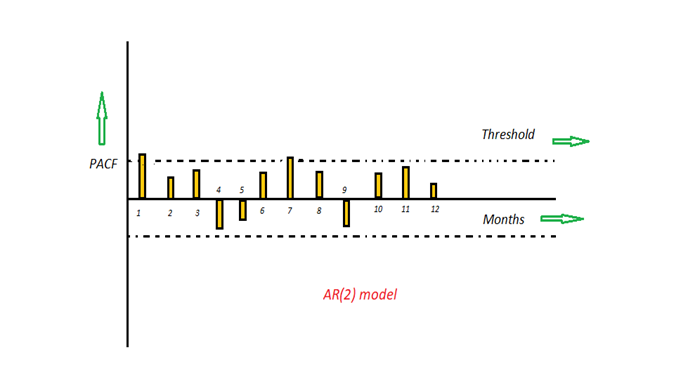Image by AuthorThe time period at t is impacted by the observation at various slots t-1, t-2, t-3, ….., t-k. The impact of previous time spots is decided by the coefficient factor at that particular period of time. The price of a share of any particular company X may depend on all the previous share prices in the time series. This kind of model calculates the regression of past time series and calculates the present or future values in the series in know as Auto Regression (AR) model.t的时间段受在t-1，t-2，t-3，…..tk不同时隙的观察影响。 先前时间点的影响由该特定时间段的系数决定。 任何特定公司X的股票价格可能取决于时间序列中所有先前的股票价格。 这种模型计算过去时间序列的回归，并以已知的自动回归(AR)模型计算序列中的当前值或将来值。
Yt = β₁* y-₁ + β₂* yₜ-₂ + β₃ * yₜ-₃ + ………… + βₖ * yₜ-ₖ Yt =β₁*y-₁+β2 *yₜ-_2+β₃*yₜ-₃+…………+βₖ*yₜ-ₖ
Consider an example of a milk distribution company that produces milk every month in the country. We want to calculate the amount of milk to be produced current month considering the milk generated in the last year. We begin by calculating the PACF values of all the 12 lags with respect to the current month. If the value of the PACF of any particular month is more than a significant value only those values will be considered for the model analysis. 考虑一个牛奶分销公司的例子，该公司每月在该国生产牛奶。 考虑到去年产生的牛奶，我们要计算当月的牛奶产量。 我们首先计算相对于当月的所有12个滞后的PACF值。 如果任何特定月份的PACF值大于显着值，则仅将这些值考虑用于模型分析。
For e.g in the above figure the values 1,2, 3 up to 12 displays the direct effect(PACF) of the milk production in the current month w.r.t the given the lag t. If we consider two significant values above the threshold then the model will be termed as AR(2). 例如，在上图中，值1,2、3到12表示给定滞后t时当月的牛奶产量的直接影响(PACF)。 如果我们认为高于阈值的两个有效值，则该模型将被称为AR(2)。
MA(移动平均值)模型
(MA (Moving Average) Model)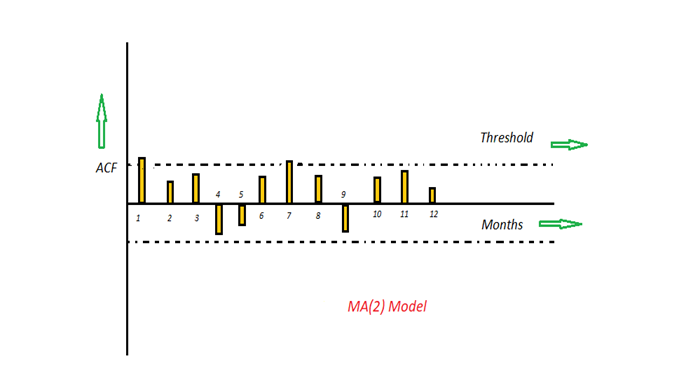Image by AuthorThe time period at t is impacted by the unexpected external factors at various slots t-1, t-2, t-3, ….., t-k. These unexpected impacts are known as Errors or Residuals. The impact of previous time spots is decided by the coefficient factor α at that particular period of time. The price of a share of any particular company X may depend on some company merger that happened overnight or maybe the company resulted in shutdown due to bankruptcy. This kind of model calculates the residuals or errors of past time series and calculates the present or future values in the series in know as Moving Average (MA) model.t的时间段受各种时隙t-1，t-2，t-3，…..tk中意外的外部因素的影响。 这些意外的影响称为“错误或残差”。 先前时间点的影响由该特定时间段的系数因子α决定。 任何特定公司X的股票价格可能取决于在一夜之间发生的某些公司合并，或者该公司可能因破产而倒闭。 这种模型可以计算过去时间序列的残差或误差，并以已知的移动平均(MA)模型来计算序列中的当前值或将来值。
Yt = α₁* Ɛₜ-₁ + α₂ * Ɛₜ-₂ + α₃ * Ɛₜ-₃ + ………… + αₖ * Ɛₜ-ₖ Yt =α₁*Ɛₜ-₁+α2*Ɛₜ-²+α₃*Ɛₜ-₃+…………+αₖ*Ɛₜ-ₖ
Consider an example of Cake distribution during my birthday. Let's assume that your mom asks you to bring pastries to the party. Every year you miss judging the no of invites to the party and end upbringing more or less no of cakes as per requirement. The difference in the actual and expected results in the error. So you want to avoid the error for this year hence we apply the moving average model on the time series and calculate the no of pastries needed this year based on past collective errors. Next, calculate the ACF values of all the lags in the time series. If the value of the ACF of any particular month is more than a significant value only those values will be considered for the model analysis. 考虑一下我生日那天分发蛋糕的例子。 假设您的妈妈要您带糕点参加聚会。 每年您都会错过判断参加聚会的邀请数并最终按照要求养成或多或少的蛋糕的机会。 实际和预期的差异会导致错误。 因此，您要避免今年的误差，因此我们将移动平均模型应用于时间序列，并根据过去的集体误差计算今年所需的糕点数量。 接下来，计算时间序列中所有滞后的ACF值。 如果任何特定月份的ACF值大于显着值，则仅将这些值考虑用于模型分析。
For e.g in the above figure the values 1,2, 3 up to 12 displays the total error(ACF) of count in pastries current month w.r.t the given the lag t by considering all the in-between lags between time t and current month. If we consider two significant values above the threshold then the model will be termed as MA(2). 例如，在上图中，值1,2、3(最多12)显示了糕点在当月的总误差(ACF)，其中给定的滞后t是考虑了时间t和当月之间的所有中间滞后。 如果我们认为高于阈值的两个有效值，则该模型将称为MA(2)。
ARMA(自动回归移动平均线)模型
(ARMA (Auto Regressive Moving Average) Model)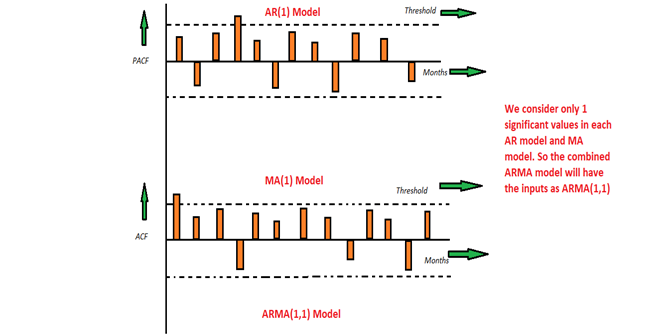Image by AuthorThis is a model that is combined from the AR and MA models. In this model, the impact of previous lags along with the residuals is considered for forecasting the future values of the time series. Here β represents the coefficients of the AR model and α represents the coefficients of the MA model.此模型是AR和MA模型的组合。 在此模型中，考虑了先前滞后的影响以及残差，以预测时间序列的未来值。 这里，β代表AR模型的系数，α代表MA模型的系数。
Yt = β₁* yₜ-₁ + α₁* Ɛₜ-₁ + β₂* yₜ-₂ + α₂ * Ɛₜ-₂ + β₃ * yₜ-₃ + α₃ * Ɛₜ-₃ +………… + βₖ * yₜ-ₖ + αₖ * Ɛₜ-ₖ Yt =β₁*yₜ-₁+α₁*Ɛₜ-₁+β2 *yₜ-²+α2 *Ɛₜ-_2+β₃*yₜ-₃+α₃*Ɛₜ-₃+…………+βₖ*yₜ-ₖ+αₖ *Ɛₜ-ₖ
Consider the above graphs where the MA and AR values are plotted with their respective significant values. Let's assume that we consider only 1 significant value from the AR model and likewise 1 significant value from the MA model. So the ARMA model will be obtained from the combined values of the other two models will be of the order of ARMA(1,1). 考虑上面的图，其中MA和AR值分别以其有效值绘制。 假设我们只考虑AR模型的1个有效值，同样考虑MA模型的1个有效值。 因此，将从其他两个模型的组合值获得ARMA模型，其数量级将为ARMA(1,1)。
ARIMA(自回归综合移动平均线)模型
(ARIMA (Auto-Regressive Integrated Moving Average) Model)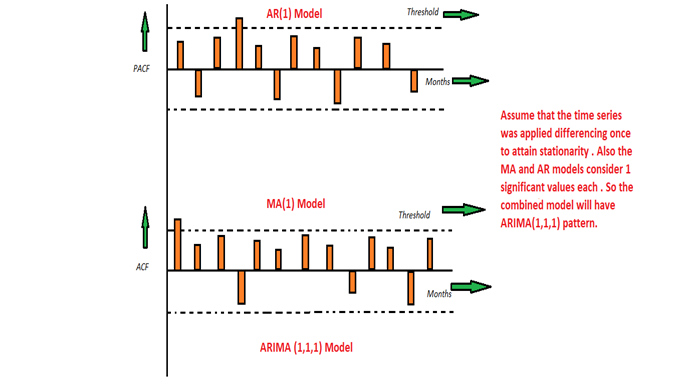Image by AuthorWe know that in order to apply the various models we must in the beginning convert the series into Stationary Time Series. In order to achieve the same, we apply the differencing or Integrated method where we subtract the t-1 value from t values of time series. After applying the first differencing if we are still unable to get the Stationary time series then we again apply the second-order differencing.我们知道，为了应用各种模型，我们必须在开始时将序列转换为平稳时间序列。 为了达到相同的目的，我们应用了微分或积分方法，其中从时间序列的t值中减去t-1值。 在应用一次微分之后，如果我们仍然无法获得固定时间序列，那么我们将再次应用二次微分。
The ARIMA model is quite similar to the ARMA model other than the fact that it includes one more factor known as Integrated( I ) i.e. differencing which stands for I in the ARIMA model. So in short ARIMA model is a combination of a number of differences already applied on the model in order to make it stationary, the number of previous lags along with residuals errors in order to forecast future values. ARIMA模型与ARMA模型非常相似，只不过它包含一个称为集成(I)的因子，即在ARIMA模型中代表I的差分。 因此，简而言之，ARIMA模型是为了使模型变得平稳而已应用到模型上的多个差异的组合，其中先前的滞后次数与残差误差一起用于预测未来值。
Consider the above graphs where the MA and AR values are plotted with their respective significant values. Let's assume that we consider only 1 significant value from the AR model and likewise 1 significant value from the MA model. Also, the graph was initially non-stationary and we had to perform differencing operation once in order to convert into a stationary set. Hence the ARIMA model which will be obtained from the combined values of the other two models along with the Integral operator can be displayed as ARIMA(1,1,1). 考虑上面的图，其中MA和AR值分别以其有效值绘制。 假设我们只考虑AR模型的1个有效值，同样考虑MA模型的1个有效值。 而且，该图最初是非平稳的，我们必须执行一次微分运算才能转换为平稳集。 因此，将从其他两个模型的组合值与积分算子一起获得的ARIMA模型可以显示为ARIMA(1,1,1)。
结论：
(Conclusion :)
All these models give us an insight or at least close enough prediction about any particular time series. Also, it depends on the users that which model perfectly suffices their needs. If the chances of error rate are less in any one model compared to other models then it's preferred that we choose the one which gives us the closest estimation. 所有这些模型为我们提供了关于任何特定时间序列的见识或至少足够接近的预测。 同样，取决于用户的是哪种型号完全可以满足他们的需求。 如果在任何一个模型中错误率的机会都比其他模型少，那么我们最好选择一个给出最接近估计值的模型。
Hope this article helps you to understand things better !! 希望本文能帮助您更好地理解！！
翻译自: https://towardsdatascience.com/time-series-models-d9266f8ac7b0时间序列模型使用流程
展开全文python 机器学习 人工智能 java 算法
• 时间序列建模的基本步骤 时间序列建模的基本步骤 习题 时间序列经典教材推荐 时间序列模型 （一）：模型概述 ...时间序列模型 （六）：平稳时间序列模型 ：自回归AR 、移动平均 MA 、ARMA 模型 时间序列模...
• 时间序列模型是什么呢？时间序列模型是按时间顺序排列的，随时间变化且相互关联的数据序列。可通过对预测目标自身时间序列的预处理，来研究其变化趋势。时间序列模型常见用途时间序列可以探索现象发展变化的规律，对...
•数据分析
• 时间序列的其它博文系列： 时间序列模型 （一）：模型概述 ...时间序列模型 （六）：平稳时间序列模型 ：自回归AR 、移动平均 MA 、ARMA 模型 时间序列模型 （七）： 时间序列建模的基本步骤 目录 移动平均法 ...
• 作者| 追光者研究| 机器学习与时间序列出品 | AI蜗牛车本篇主要讲解AR，ARMA，ARIMA等传统时间序列模型，包括具体代码操作。并附讲时间序列的一些基础知识点，如果有基础的可以直接跳到模型部分。1. 时间序列的平稳...
• 精心编写的R语言时间序列模型（主要为Arima模型），程序里我给出了很详细的备注，相信即使是编程小白或者统计小白也可以看懂。内容包含数据集
• ____ ____ ____ (R)/__ / ____/ / ____/___/ / /___/ / /___/ 13.1 Statistics/Data Analysis此次系列文章的主题是通过Stata软件来分析时间序列的平稳和非平稳关系，以及如何通过Stata软件来进行不同时间序列模型的...
• 上期我们学习到Eviews时间序列模型预测，这期就常见问题进行解答。01ARIMA(pnq)模型含义？答: ARIMAAuto模型主要包含三个部分,其中AR表示 regression,即自回归模型;I表示 integration,即单整阶数;m表示 moving ...
•Python
•研究论文
• 时间序列模型及其性质为了能够描述时间序列之间的内在联系及其发展规律，需要建立时序模型，它能够有效的用于时间序列的预测和控制分析。目前，常用的时间序列模型有自回归模型（Auto Regressive Model，简称 AR ...
• 上几期小统带大家一起学习了Eviews经典线性模型，这期我们开始学习时间序列模型。01 ARIMA简介ARIMA，差分自回归滑动平均模型，又称求自回归滑动平均模型，是时间序列预测分析方法之一。ARIMA(p,d,q)中，AR是“自......